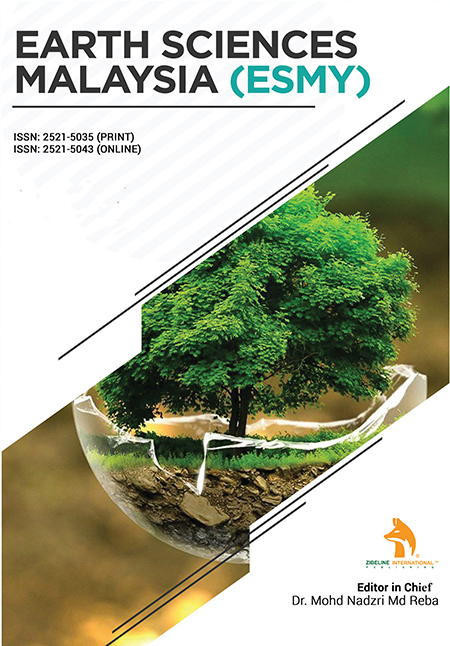# Earth Science Malaysia (ESMY)

### COMPARATIVE ANALYSIS OF STATIC SHEAR MODULUS AND DYNAMIC SHEAR MODULUS DETERMINED BY GEOPHYSICAL AND GEOTECHNICAL INVESTIGATION

December 27, 2021 Posted by dgnoraina In Earth Science Malaysia (ESMY)

## ABSTRACT

### COMPARATIVE ANALYSIS OF STATIC SHEAR MODULUS AND DYNAMIC SHEAR MODULUS DETERMINED BY GEOPHYSICAL AND GEOTECHNICAL INVESTIGATION

Journal: Earth Science Malaysia (ESMY)

Udota S. Benjamin, Tamunobereton-ari I., Horsfall I. Opiriyabo, Mogaba P

This is an open access article distributed under the Creative Commons Attribution License CC BY 4.0, which permits unrestricted use, distribution, and reproduction in any medium, provided the original work is properly citedDue to the occurrence of earth tremors which leads to the vibrations of foundations and perhaps failure of buildings and roads, it is therefore important to understand and have knowledge of the geomechanical soil properties for foundation design, assessment of risks and suggestion of mitigation plans in engineering structures and road construction. A total of 3 boreholes were drilled with the Standard Penetration Test (SPT) performed and Downhole Seismic Test (DST) carried out in the boreholes located within Assa to investigate the Geomechanical soil properties in the area. For the geophysical survey, the downhole seismic test was carried out to determine the P-wave and S-wave. The results were processed using the generalized reciprocal method (GRM) with the Seisimager program. The results of soil dynamic modulus (shear, young and bulk modulus) and Poisson ratio recorded from DST conducted in BH1, BH2 and BH3 ranges from 7300 KPa to 72390 KPa, 0.31 to 0.41 for the Poisson ratio. Meanwhile, soil static modulus and Poisson’s ratio recorded from SPT conducted in BH1, BH2 and BH3 ranges from 2520 to 44687.0 KPa, 0.20 to 0.55 for the Poisson ratio respectively. The results of this study have shown that there is a wide variation between geomechanical properties derived from geotechnical investigations (static properties) and geophysical investigations (dynamic properties). Based on depth trend analysis, the dynamic and static soil elastic properties all increases with depth. Generally, the dynamic soil properties were significantly higher than the static elastic properties. At shallow depths (<12.0 m), the difference between static and dynamic soil modulus was relatively small, but increased with increasing depth. Meanwhile, the difference between static and dynamic Poisson ratio was high at shallow depth and it decreased with increased depths where they almost overlap. Correlation between the derived static and dynamic properties all revealed positive correlation trends. The strength of the correlation was highest for young modulus (r=0.87) which was closely followed by the shear modulus (r=0.63). Meanwhile, Poisson ratio (r=0.40) and bulk modulus (r=0.23) revealed weak positive correlation trends. The regression models generated from this study were used to derive static elastic properties and compared with the static properties obtained from geotechnical investigation thereby deriving the equations Dynamic Shear Modulus = (1.4207 x Static Shear Modulus) + 5022, Dynamic Young Modulus = (2.0241 x static young modulus) + 5054.8, Dynamic Bulk Modulus = (1.7852 x static bulk modulus) + 15458, Dynamic Poisson’s ratio = (0.1812 x Static Poisson’s ratio) + 0.3154. The results showed fairly good match between static (geotechnical) shear modulus and static (from regression model) shear modulus, static (geotechnical) young modulus and static (from regression model) young modulus. There was no good match obtained for bulk modulus and Poisson ratio generally, except at shallow depth (< 12 m depth) where Poisson ratio revealed a good match.

 Pages 01-10 Year 2022 Issue 1 Volume 6